# Meddling with Mechanics problems!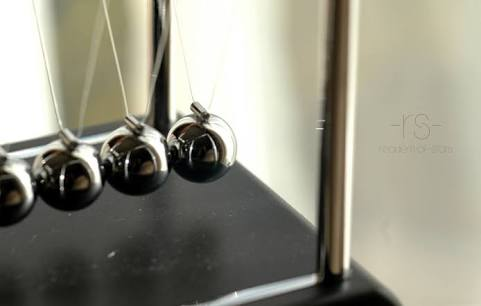Greetings Mechanics Lovers!

Since many people are starting their preparations for entering into most prestigious institute of India,(IITs),lets start a Mechanics Marathon.This Marathon will be topic-oriented,i.e. we will try to post good questions of certain topics for first three weeks.This note will help us to improve our problem solving strategy.

Rules are simple as other contest/marathons.

•I'll post two problems.If anybody solves any one of the two problems,s/he should post next problem within 3 hours.

•There will be max 2 unsolved problems at a time.

•Make sure you know how to solve your own problem before posting it, in case no one else is able to solve it within 24 hours. Then, you must post the solution and you have the right to post a new problem.

•It is NOT compulsory to post original problems. But make sure it has not been posted on brilliant.Also please don't post HC Verma questions or DC Pandey questions.

•There should be only one question. No sub questions allowed.No parts. Only one answer should be there.

•The scope of the problems is Jee-Advance and NSEP.(mechanics only).

Topics for first three weeks:

• Vectors

• Kinematics

• Laws of motion

• Work Energy and Power

• Circular Motion

• Center of mass.

Post only the problem and it's solution here. Extremely relevant comments are allowed.You are allowed to discuss solutions and problems here.

Format your proof as follows:

Solution to Problem (Insert Problem no here)

[Post your solution here]


And put your Problem in a new thread following the format :

Problem (Insert Problem no here)

[Post your problem here]


Remember to reshare this note so it goes to everyone out there. And above all else, have fun!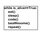Note by Harsh Shrivastava
4 years, 11 months ago

This discussion board is a place to discuss our Daily Challenges and the math and science related to those challenges. Explanations are more than just a solution — they should explain the steps and thinking strategies that you used to obtain the solution. Comments should further the discussion of math and science.

When posting on Brilliant:

• Use the emojis to react to an explanation, whether you're congratulating a job well done , or just really confused .
• Ask specific questions about the challenge or the steps in somebody's explanation. Well-posed questions can add a lot to the discussion, but posting "I don't understand!" doesn't help anyone.
• Try to contribute something new to the discussion, whether it is an extension, generalization or other idea related to the challenge.
• Stay on topic — we're all here to learn more about math and science, not to hear about your favorite get-rich-quick scheme or current world events.

MarkdownAppears as
*italics* or _italics_ italics
**bold** or __bold__ bold
- bulleted- list
• bulleted
• list
1. numbered2. list
1. numbered
2. list
Note: you must add a full line of space before and after lists for them to show up correctly
paragraph 1paragraph 2

paragraph 1

paragraph 2

[example link](https://brilliant.org)example link
> This is a quote
This is a quote
    # I indented these lines
# 4 spaces, and now they show
# up as a code block.

print "hello world"
# I indented these lines
# 4 spaces, and now they show
# up as a code block.

print "hello world"
MathAppears as
Remember to wrap math in $$ ... $$ or $ ... $ to ensure proper formatting.
2 \times 3 $2 \times 3$
2^{34} $2^{34}$
a_{i-1} $a_{i-1}$
\frac{2}{3} $\frac{2}{3}$
\sqrt{2} $\sqrt{2}$
\sum_{i=1}^3 $\sum_{i=1}^3$
\sin \theta $\sin \theta$
\boxed{123} $\boxed{123}$

## Comments

Sort by:

Top Newest

PROBLEM 11

Moment of inertia of a uniform hexagonal plate about an axis LL' is I . The moment of inertia (about XX') of an equilateral uniform triangular plate of thickness double that of hexagonal plate is P . (specific gravity of triangular plate is 3 times that of hexagonal plate)

Find I/P

- 4 years, 3 months ago

Log in to reply

https://s28.postimg.org/vhjxmwknx/Untitled.png

- 4 years, 3 months ago

Log in to reply

http://tinypic.com/view.php?pic=2ludvn5&s=9#.WGoB81N96M8

- 4 years, 3 months ago

Log in to reply

file:///C:/Users/user/Downloads/Untitled.webp

visit this page for image

- 4 years, 3 months ago

Log in to reply

ans is 5

- 4 years, 3 months ago

Log in to reply

Post the next problem , a tough one!

- 4 years, 3 months ago

Log in to reply

This was very similar to a problem which appeared in our AITS 1

- 4 years, 3 months ago

Log in to reply

wasn't this one tough? :P

- 4 years, 3 months ago

Log in to reply

It was tough indeed, though I have only tried it once.

- 4 years, 3 months ago

Log in to reply

Yup correct!

- 4 years, 3 months ago

Log in to reply

Problem 10

A meteorite of mass m collides with a satellite which was oribiting around a planet of mass $M$ in a circular orbit of radius R . Due to collision, the meteorite sticks to satellite and satellite is seen to have entered an orbit in which its minimum distance from the planet is $\frac{R}{2}$. If mass of the satellite is is 10 times that of the meteorite , What was the velocity of the meteorite before collision ? (edit : Assume velocity of meteorite was initially perpendicular to that of the satellite)

- 4 years, 3 months ago

Log in to reply

Its a simple energy , momentum and angular momentum conservation problem

on solving v turns out to be sqrt (58GM/R)

- 4 years, 3 months ago

Log in to reply

Post the next problem!

- 4 years, 3 months ago

Log in to reply

I might I have done a serious calculation mistake coz I also did the same.

- 4 years, 3 months ago

Log in to reply

Yes :P.......Plz post the next problem

- 4 years, 3 months ago

Log in to reply

Is the answer sqrt(288GM/R)?

- 4 years, 3 months ago

Log in to reply

no

- 4 years, 3 months ago

Log in to reply

Is this dead? Shall I participate

- 4 years, 3 months ago

Log in to reply

If you want you may post problems here and I will participate here.So post the next problem !

- 4 years, 3 months ago

Log in to reply

Can u derive the equation of trajectory of each particle in the Irodov question no 1.12?

- 4 years, 3 months ago

Log in to reply

Is the contest dead?

- 4 years, 10 months ago

Log in to reply

Oh, sad. But no worries! I'll be coming up with an Electromagnetism contest on Monday, be sure to take part!

- 4 years, 10 months ago

Log in to reply

Yeah :P . No more activity as we all are quite busy with our class 12th!

- 4 years, 10 months ago

Log in to reply

Problem 9

A metal rod is of length $1m$ is clamped at points $5cm$ and $15 cm$ from either ends. Find the minimum frequency of natural longitudinal oscillations of rod.

Given :

• Young's modulus of rod $= 1.6 \times 10^{11} Pa$

• Mass per unit volume $\rho = 2500 kgm^{-3}$

- 4 years, 10 months ago

Log in to reply

I did it like this. The particles at ends of the rod are the ones which will oscillate with max amplitude(antinodes). In case of minimum frequency , we are looking to maximize wavelength. Hence consider the case when antinode is at one end and first node is at the hinge 5cm from it.(This also takes care of a node being at the other point that is hinged) . hence max wavelength is 20cm. Thus min frequency is $40,000 Hz$

- 4 years, 3 months ago

Log in to reply

Awesome! We got a new problem poster!

Post the next problem please!

- 4 years, 3 months ago

Log in to reply

correct!

- 4 years, 3 months ago

Log in to reply

pic

- 4 years, 10 months ago

Log in to reply

Rod is clamped at a distance 5cm from one end and 15 cm from other end. Its easily understandable i guess.

- 4 years, 10 months ago

Log in to reply

yep it is but i thought you were not able to do as guided by me.

- 4 years, 10 months ago

Log in to reply

yes i didn't understand the method u said to post the pic.

- 4 years, 10 months ago

Log in to reply

be on slack

- 4 years, 10 months ago

Log in to reply

I need to go to coaching right now. so talk to you later

- 4 years, 10 months ago

Log in to reply

ok bye today i have coaching too from 2-30 so better talk at 9-00

- 4 years, 10 months ago

Log in to reply

Problem 8: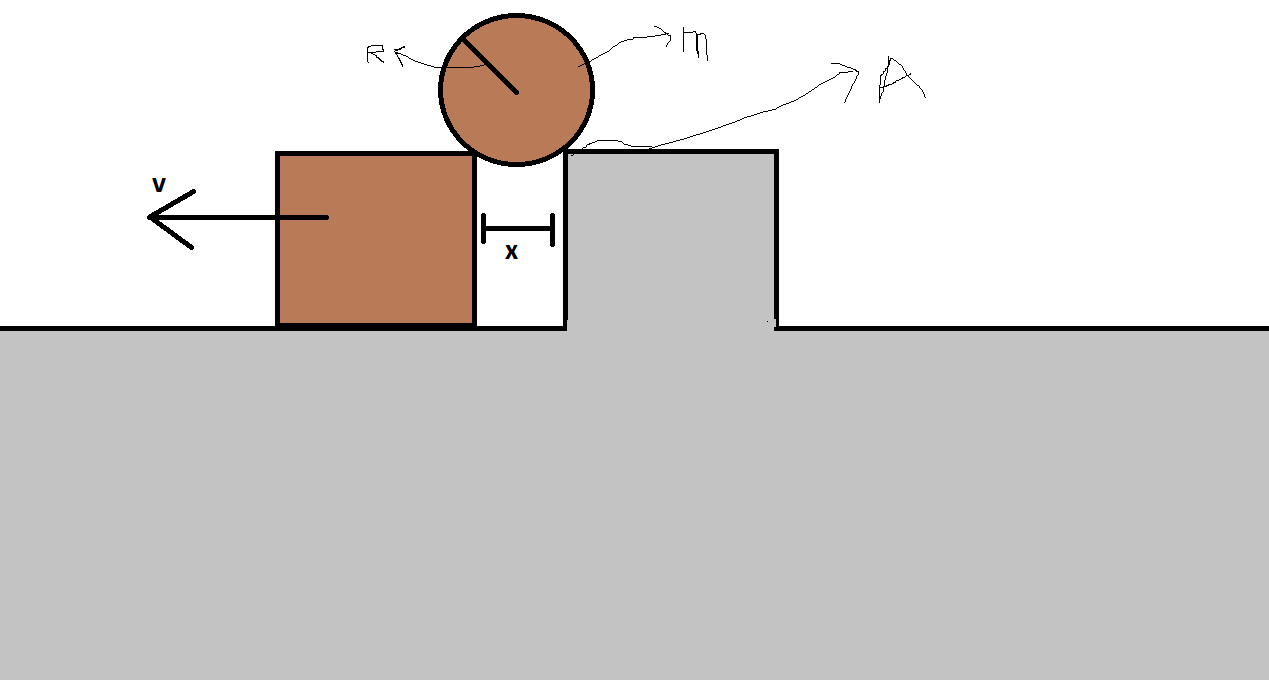A cylinder of mass $m$ and radius $R$ rest on two supports in the same height .One support is stationery while the other slides from the cylinder at velocity $v$. Determine the force of normal pressure $N$ by cylinder at stationery support when $x=R\sqrt{2}$.

- 4 years, 10 months ago

Log in to reply

Solution to problem 8:

As the cylinder remains in contact with the stationary support it does circular motion about that point. As the cylinder is in contact with the block so the velocity of block on the normal of point of contact (which passes through center of cylinder) should be equal to that of cylinder.

$\Rightarrow v_c = \dfrac{v}{\sqrt{2}}$

As the block does circular motion the forces perpendicular to the point of contact provide the required centripetal force.

$mg \cos {45^{\circ}} - N = \dfrac{mv_c ^2}{R}$

$N = \dfrac{mg}{\sqrt{2}} - \dfrac{mv^2}{2R}$

- 4 years, 10 months ago

Log in to reply

go and post the next q

- 4 years, 10 months ago

Log in to reply

How to post image. ? I need image to illustrate the question.

- 4 years, 10 months ago

Log in to reply

when will you post q

- 4 years, 10 months ago

Log in to reply

you just get the link by by copying it from publish place where you post q.

- 4 years, 10 months ago

Log in to reply

Solution to Problem 8:

The answer is $n=\frac{mg}{\sqrt{2}}-\frac{mv^2}{2R}$. Due to time constraint I can't post the complete solution. But it can be found out by balancing forces. The angle made with horizontal can be found out by basic geometry. The velocity of the cylinder is $\frac{v}{\sqrt{2}}$. This can be found out by equating components.

- 4 years, 10 months ago

Log in to reply

I can't find a good problem now, can anyone else post it?

- 4 years, 10 months ago

Log in to reply

Problem 7: A lift of total mass M kg is raised by cables from rest to rest through a height h.The greatest tension which the cables can safely bear is n Mg Newtons(n>1).Find the shortest interval of time in which the ascent can be made,in terms of n,h,g.

- 4 years, 10 months ago

Log in to reply

now this can have multiple ans depending on if cables are also attached at bottom for retardation or not i assume that the cable bring only acceleration and retardation is brought up by g only in that case i hope t(min)=root(2h/{n(n-1)g})(considering at a ht h v=0)

- 4 years, 10 months ago

Log in to reply

The latter case is correct!Well done!Post the next question please!

- 4 years, 10 months ago

Log in to reply

Hey guyz,please delete unnecessary comments as the note is getting slow.Thanks.

- 4 years, 10 months ago

Log in to reply

Problem 6 : [Original]

Take a bomb of mass m and give it a velocity $v = 10m/sec$ at an angle $\theta = 53°$with horizontal. The bomb bursts into two identical parts when it's velocity made an angle +45° with horizontal. Just after the breakdown, One of the part's velocity was found to be $6 m/sec$ in horizontal direction. (i. e. It retained it's horizontal component of velocity ) . Find the final displacement of one part with respect to other after they come to rest.

Details And Assumptions :

• The coefficient of restitution for the ball ground collision is $e = 0.4$

• The ground is smooth.

• Take $g = 10m/s^2$

- 4 years, 10 months ago

Log in to reply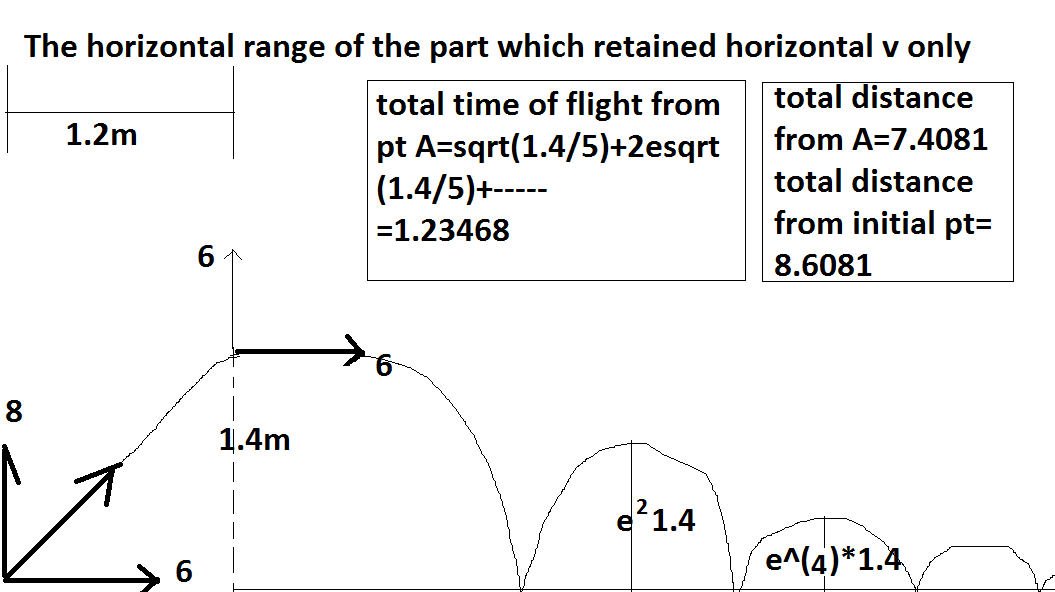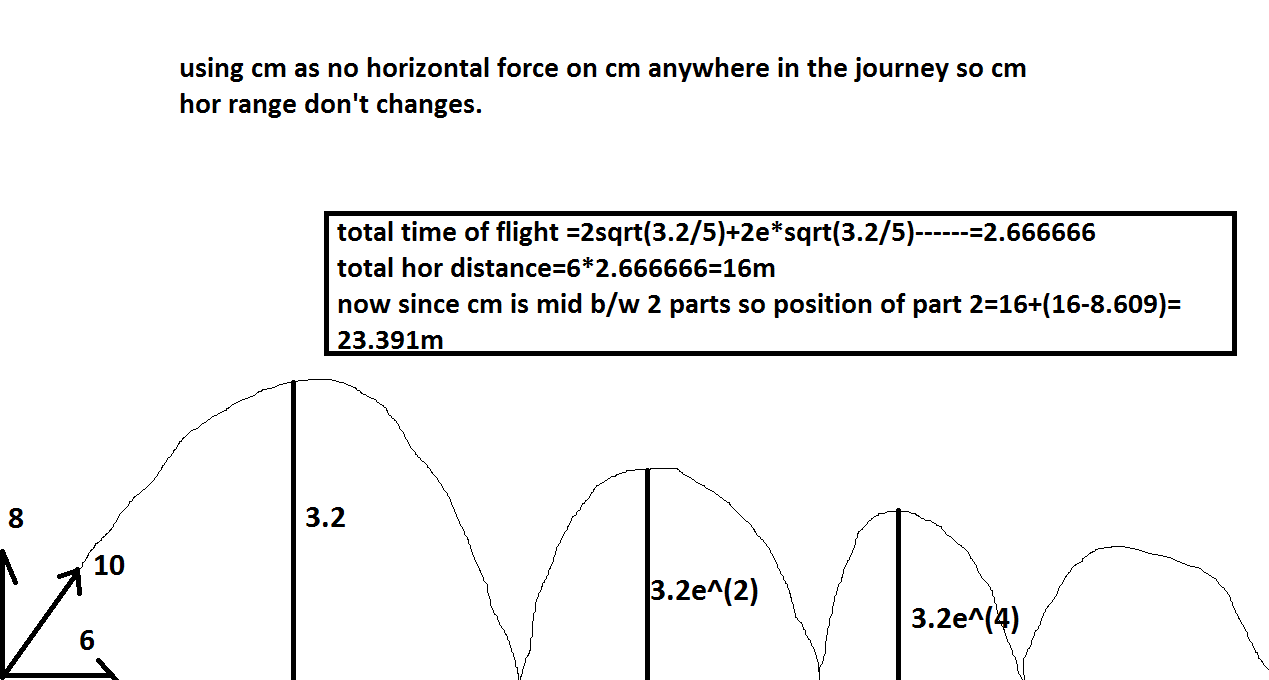- 4 years, 10 months ago

Log in to reply

this gives ans as 14.782 as suggested by @Prakhar Bindal i request to find a mistake in it??

- 4 years, 10 months ago

Log in to reply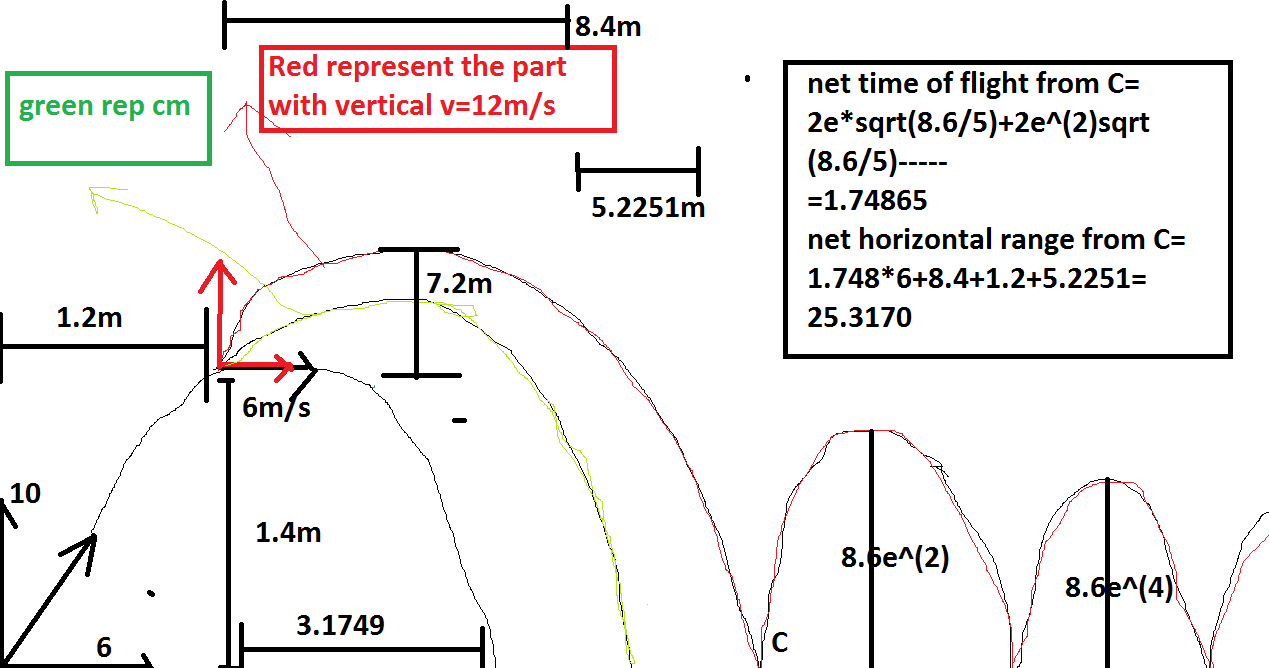- 4 years, 10 months ago

Log in to reply

this gives ans as 16.71 as suggested by @Aniket Sanghi i request to find a mistake in this.

- 4 years, 10 months ago

Log in to reply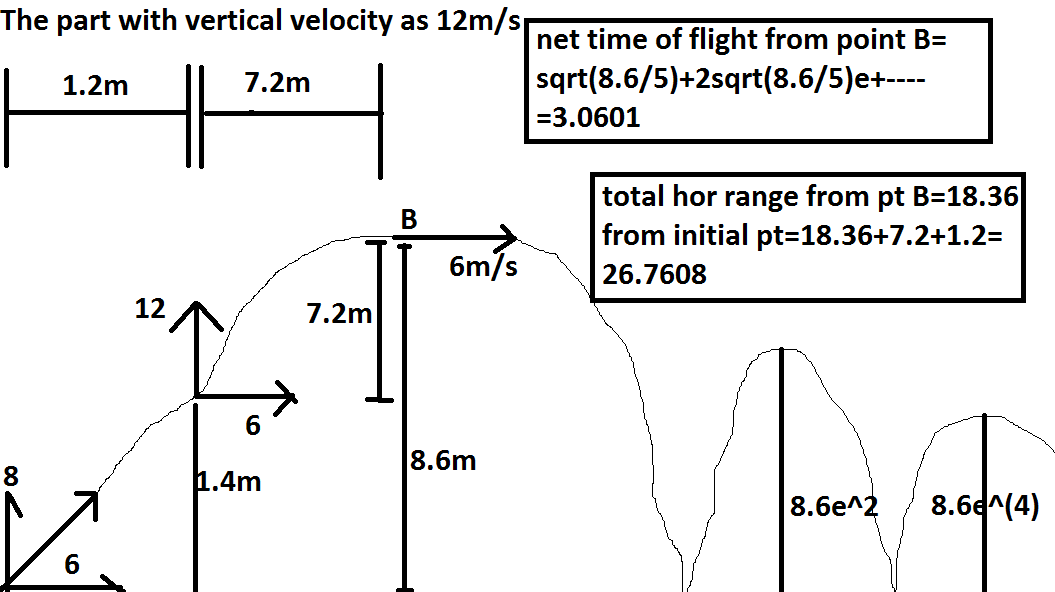- 4 years, 10 months ago

Log in to reply

this gives ans 18.1527 as mentioned by @Adarsh Kumar i would request to find a mistake in this?????

- 4 years, 10 months ago

Log in to reply

Thanx!@aryan goyat for posting this!

- 4 years, 10 months ago

Log in to reply

Go! Post the next question for now @AdarshKumar

- 4 years, 10 months ago

Log in to reply

Ohk,gimme a minute I just woke up :P.

- 4 years, 10 months ago

Log in to reply

welcome

- 4 years, 10 months ago

Log in to reply

You may post the solution and the next problem.

- 4 years, 10 months ago

Log in to reply

@Aniket Sanghi @Deeparaj Bhat please post the next question!

- 4 years, 10 months ago

Log in to reply

Problem 5

A chain of length L is placed on a smooth spherical surface of radius R with one of its ends fixed at the top of the sphere . What will be the acceleration of each element of the chain when its upper end is released

It is assumed that the length of chain is less than quarter of the sphere

- 4 years, 11 months ago

Log in to reply

Solution to problem 5 :

Take a small element dx making angle $d\theta$ with centre ( at an angle $\theta$ with horizontal.)

Check for the tangential forces acting on it you will find that only one force act on it tangentially and that is

$dm g cos\theta$ $( dm = \frac{MRd\theta}{L}$ ( where M is assumed as mass of chain which will cancel at end))

[

Edited : Sorry guys , I left one thing { Credit to Deeparaj Bhat }

There is one more tangential force , that is dT , but since tension is an internal force the integration of dT vanish .

]

This is the force on a single element , integrate it to get the total force and then divide it by mass to get acceleration , which you will get as

$a = \frac{gR}{L} ( 1 - cos(\frac{L}{R}))$

Calculations I left for you !

- 4 years, 11 months ago

Log in to reply

@Harsh Shrivastava if you can remove the barrier of topics on posting questions , I will like post my next q that will be original! And will include a combination of topics.

- 4 years, 11 months ago

Log in to reply

Is the answer a = (gR/L)(1 - cos (L/R))

- 4 years, 11 months ago

Log in to reply

Correct! . Post next question and solution

- 4 years, 11 months ago

Log in to reply

Problem 4:

The trajectory for motion of a particle in $x-y$ plane can be expressed as given below,

$e^x+e^y=e^x e^y, x,y \in \mathbb{R}$

It is given that acceleration of this particle is zero and its initial velocity in $x$ and $y$ direction are $1 \text{m/s}$ and $2 \text{m/s}$ respectively. Also if its initial coordinates can be expressed in form integers, then find the initial coordinates $(x,y)$ of the particale.

If no such coordinates exist then enter your answer as 2016.

P. S. : Original problem.

- 4 years, 11 months ago

Log in to reply

Given equation:

$1=e^{-x}+e^{-y} \\\implies 0=e^{-x}+2e^{-y} \\ (\text{differentiating wrt time } \frac{dx}{dt}=1\: \frac{dy}{dt}=2) \\\implies -1=e^{-y} \\\text{No real solution}\\\large Q. E. D.$

- 4 years, 11 months ago

Log in to reply

Can you please explain third line of you solution ?

- 4 years, 10 months ago

Log in to reply

I meant that the second line is obtained after differentiating the given equation and using the given facts.

- 4 years, 10 months ago

Log in to reply

Correct! post the next question!

- 4 years, 11 months ago

Log in to reply

Let's start with an easy one!

Problem 3: The velocity of a particle moving in the positive direction of the $x$ axis varies as $v=\alpha\sqrt{x}$, where $\alpha$ is a positive constant. Assuming that at the moment $t=0$ the particle was located at the point $x=0$, find the time dependence of the velocity and acceleration of the particle.

- 4 years, 11 months ago

Log in to reply

$v=\alpha^2t/2$ and $a=\alpha^2/2$.

We know,

$v=\frac{dx}{dt}=\alpha x^{1/2}$

$x^{-1/2}dx=\alpha dt$

$\displaystyle \int_{0}^{x}x^{-1/2}dx=\displaystyle \int_{0}^{t} \alpha dt$

$2x^{1/2}=\alpha t$

$x=\alpha^2 t^2/4$

By differentiation,

$v=\alpha^2 t/2$

$a=\alpha^2/2$

- 4 years, 11 months ago

Log in to reply

Correct.You perhaps can post the next question.

- 4 years, 11 months ago

Log in to reply

I am inviting few people to join the contest, sorry for mass taging.

@Akshay Yadav @Aniket Sanghi @Adarsh Kumar @Chinmay Sangawadekar @Vaibhav Prasad @Kalash Verma @Hrishik Mukherjee. @Nihar Mahajan @Prakhar Bindal @Swapnil Das @Rajdeep Dhingra @Shreya R @milind prabhu @Kaustubh Miglani @Vighnesh shenoy

- 4 years, 11 months ago

Log in to reply

Thanks for inviting :)

- 4 years, 11 months ago

Log in to reply

Reshare the note so that it may reach out to others.Thanks!

- 4 years, 11 months ago

Log in to reply

Problem 2: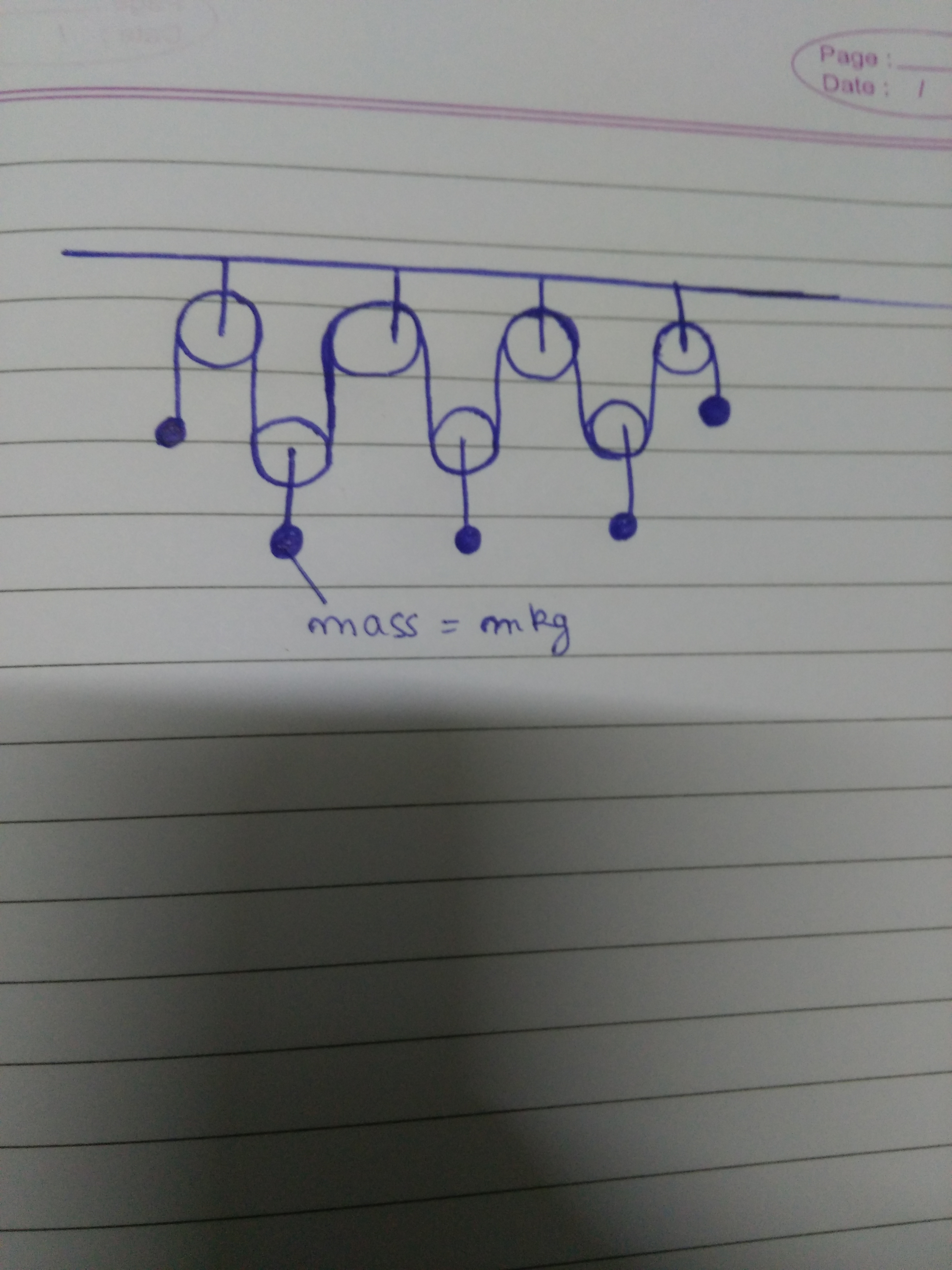5 equal masses hang as shown in the figure.Find acceleration of each body.Assume pulleys to be frictionless,light and string is inextensible.

- 4 years, 11 months ago

Log in to reply

Its an elementary problem on virtual work method

By Symmetry Of forces acceleration of left most and right most will be same and acceleration of middle 3 will be same.

Apply Virtual work method

Call acceleration of extremum's to be a1 and middle ones to be a2

You get

2Ta1 = 6Ta2

a1 = 3a2

Apply Newton's second for two blocks

mg-T =ma1

2T-mg = ma2

Rest is algebra which is left as an exercise for reader

On Solving a1 = 3g/7 and a2 = g/7

- 4 years, 11 months ago

Log in to reply

@Prakhar Bindal Hello Prakhar. Could you please tell me where I could learn about this Virtual Work method, especially on how to apply it for JEE problems? I had never heard of it before and it seems quite interesting! Thanks in advance!

- 4 years, 10 months ago

Log in to reply

Hey hello @Ishan Dasgupta Samarendra . Long time no see . how are you?

About your question actually i was taught this by my teacher in Laws Of Motion in FIITJEE .

I Am giving you some links for learning.

https://books.google.co.in/books?id=imp4CgAAQBAJ&pg=SA6-PA34&lpg=SA6-PA34&dq=virtual+work+method+JEE&source=bl&ots=ka5Dc74yLI&sig=JJ7L4LQfIFhgeWR0dOg_D95gJrw&hl=en&sa=X&ved=0ahUKEwiwjMHy-pnNAhWEk5QKHeykDjwQ6AEIGzAA#v=onepage&q=virtual%20work%20method%20JEE&f=false

https://www.youtube.com/watch?v=lsHXK8nlLBs&feature=youtu.be

- 4 years, 10 months ago

Log in to reply

Thank you very much for the links. I'll go through them right away! I'm fine, thanks! Just buried under a huge amount of work, and hence sadly I've got no spare time for Brilliant. What about you? However I do see most of the problems you post on Chemistry; I really like them. Aachchaa, which books do you study from for P, C and M? As in theory as well as practice problems.Lastly, which center are you at FIITJEE? I seem to recall seeing your name once somewhere...

- 4 years, 10 months ago

Log in to reply

@Prakhar Bindal @Ishaan Dasgupta Samarendra

How did you guyz managed your time in class 11?

How many hours did you studied?

Please guide me.Thanks!

- 4 years, 10 months ago

Log in to reply

I too am not the right person to ask. However one of the major mistakes I made in class 11 was that I never planned by which dates to finish which chapters. One should always have a countdown so that one knows exactly how far behind/ahead one is. For example, say you practise questions for Physics from sources A,B and C. Then you must have days scheduled by when you'll finish A, B and C respectively. That way you'll know whether you have been working the way you had to, or not.

- 4 years, 10 months ago

Log in to reply

I Think i am not the right person to be asked . you must contact the people who have given JEE This year

@Mayank Singh @Deeparaj Bhat @Abhineet Nayyar

- 4 years, 10 months ago

Log in to reply

Thanks! . I Am bad at chemistry :P.

You too know the amount of work fiitjee people give :P . so i am left with very less amount of time to complete other books but still i have some

Maths---> I refer Amit Mohan Agarwal's Skills in mathematics Series by Arihant

Physics----> I Have HC Verma for theory and DC Pandey for problems . i too have irodov but i hardly pick it up

Chemistry----> Physical - NCERT For Theory and RC Mukherjee for problems

Organic- Solomon's for theory and Himanshu Pandey for problems

Inorganic---> :P . i have JD Lee but i haven't opened it from last 6 months!

I Am from FIITJEE East Delhi centre (Laxmi Nagar) . Where are you from ? . where did you saw my name?

- 4 years, 10 months ago

Log in to reply

Oh, I too have many of the books you use! Anyway thanks very much for telling me ! I'm from the South Delhi Center. I must have seen your name in some AIITS result or somewhere else. i really can't remember.

- 4 years, 10 months ago

Log in to reply

Might be. !

- 4 years, 10 months ago

Log in to reply

Fun fact: Himanshu Pandey sir is my chemistry teacher(for inorganic and organic).

- 4 years, 10 months ago

Log in to reply

Cool! . he must be a master in organic chemistry

- 4 years, 10 months ago

Log in to reply

Problem 1:

Two particles, 1 and 2, move with constant velocities v1 and v2. At the initial moment their radius vectors are equal to r1 and r2. How must these four vectors be interrelated for the particles to collide?

- 4 years, 11 months ago

Log in to reply

Let the particles collide at point $P$, whose position vector is ${ r }_{ 3 }$. Let the time taken be $t$. By the vector laws of addition, we get

${ r }_{ 3 }={ { r }_{ 1 } }+{ v }_{ 1 }t\\ { r }_{ 3 }={ r }_{ 2 }+{ v }_{ 2 }t$

$\therefore$. ${r_1}- {r_2} = ({v_2} -{v_1})t$

$\large t$= $\large\frac{({r_1} - {r_2})}{({v_2}-{v_1})}$
From the system of equations,we get $\large \frac{({r_1} - {r_2})}{|({r_1}-{r_2})|} = \frac{({v_2} -{v_1})}{(|{v_2}-{v_1}|)}$

By intuition, we can tell that the particles $1$ and $2$ collide when velocity of the second is directed towards the first. In that case, the velocity vectors turn parallel to the position vectors, thus making their unit vectors equal.

- 4 years, 11 months ago

Log in to reply

Nice solution (+1) :).

Don't you think the velocities, in addition of being parallel, must be not be equal to each other in case $r_{1} ≠ r_{2}$ ?

- 4 years, 10 months ago

Log in to reply

Thanks For The Invite.Is the answer to this question v2 vector-v1vector/mod(v2 vector -v1 vector)=r2vector-r1vector/mod(r2vector-r1 vector)

- 4 years, 11 months ago

Log in to reply

×

Problem Loading...

Note Loading...

Set Loading...